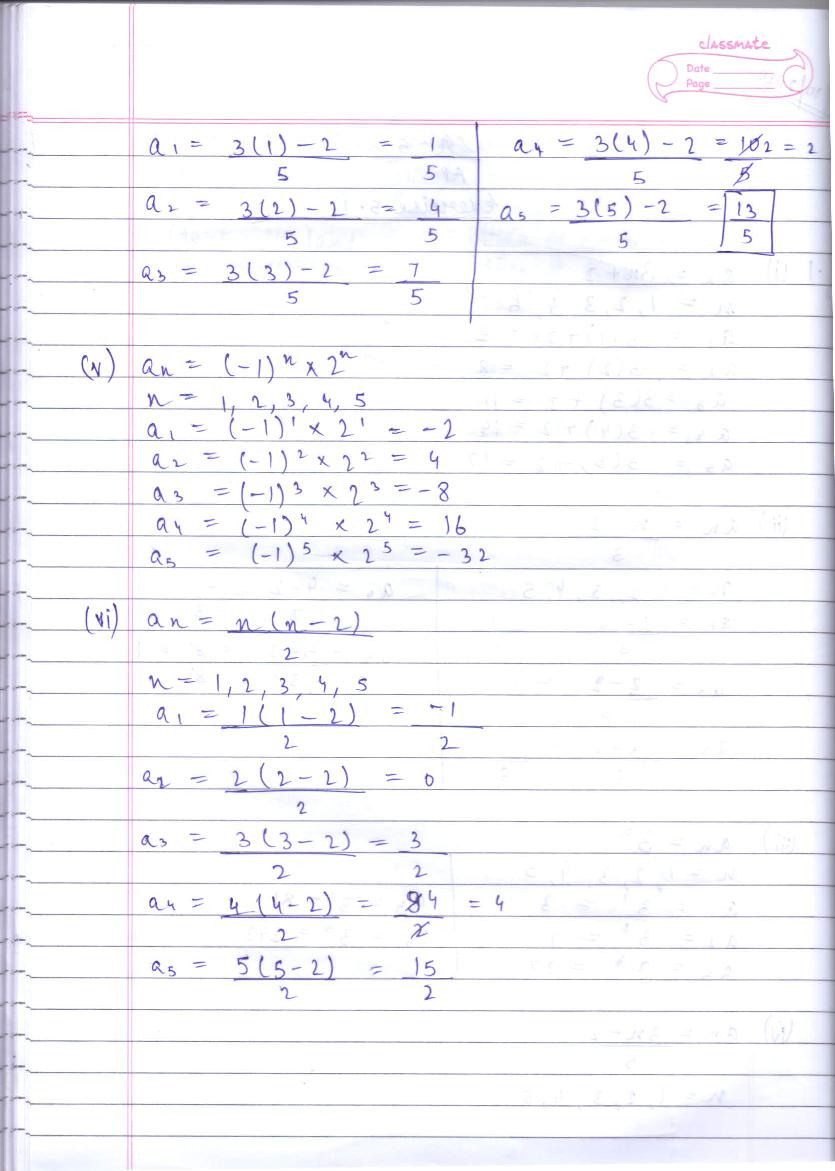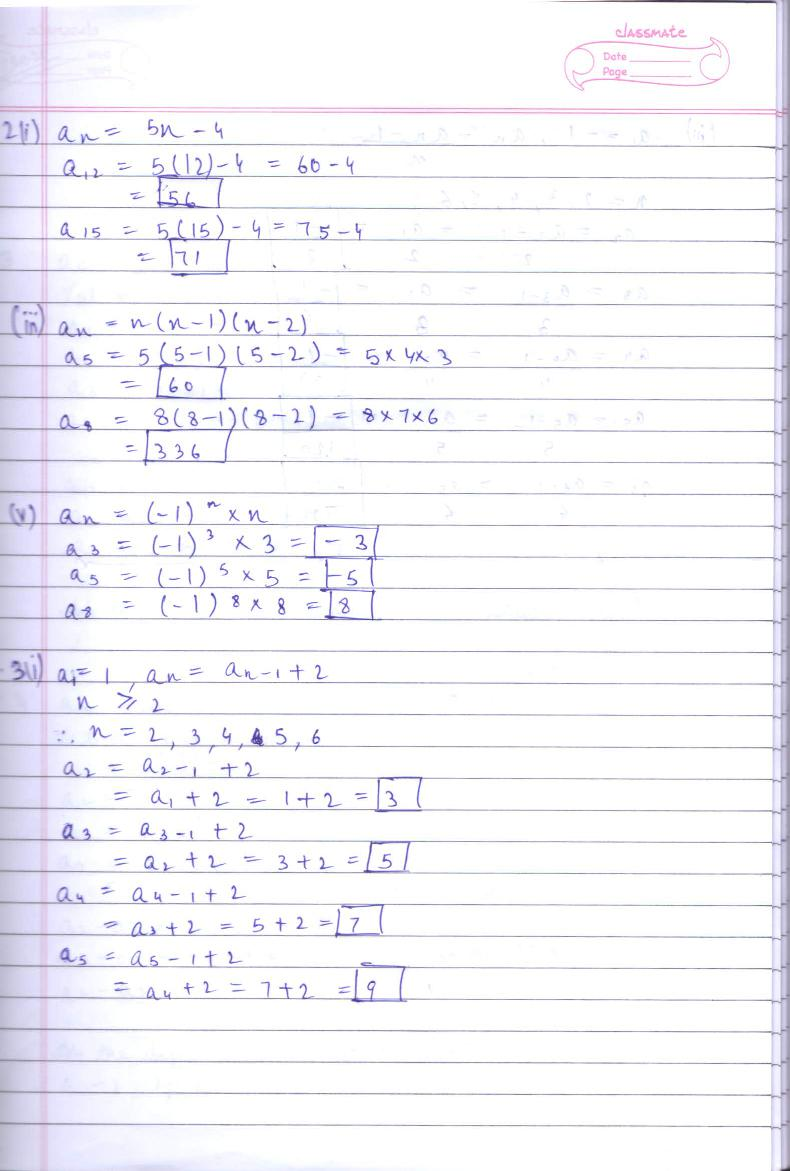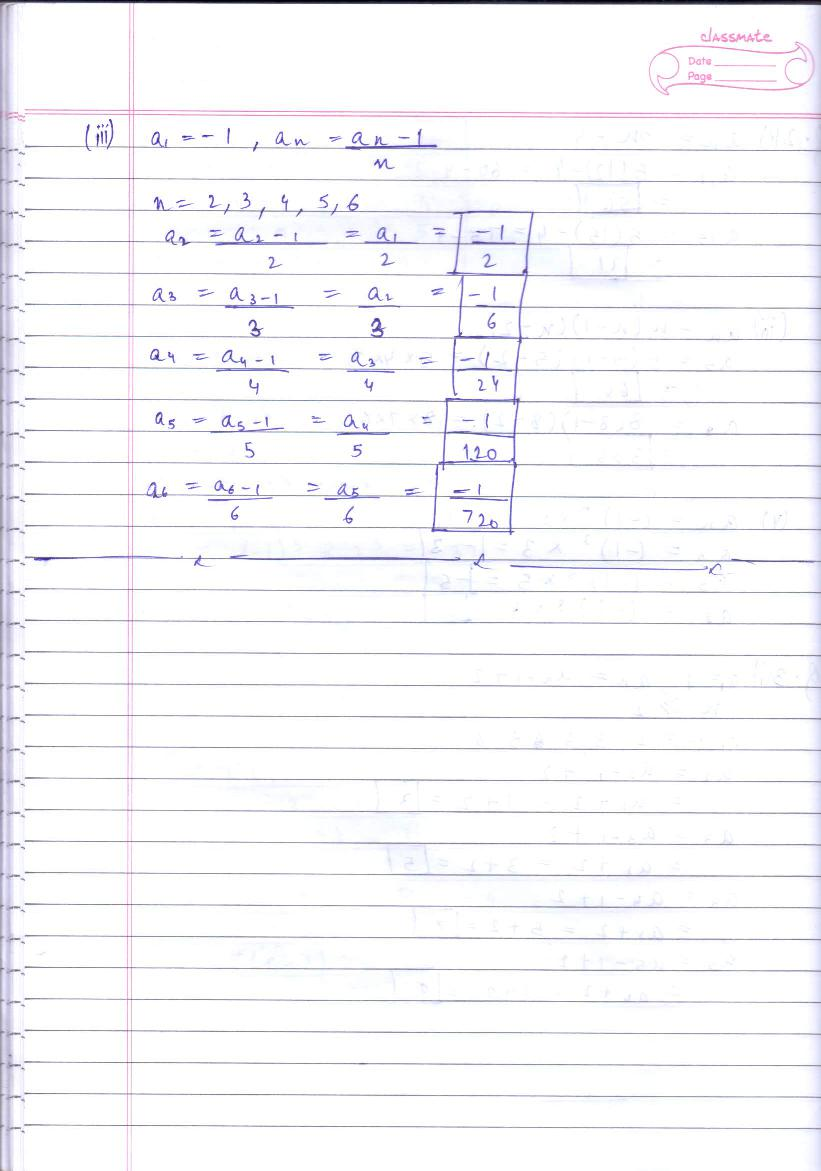# RD Sharma Solutions Class 10 Chapter 5 Arithmetic Progressions Exercise 5.1

Here you can get free RD Sharma Solutions for Class 10 Maths Chapter 5 Arithmetic Progressions Exercise 5.1. All RD Sharma Book Solutions are given here exercise wise for the chapter Arithmetic Progressions. RD Sharma Solutions are helpful in the preparation of several school level, graduate and undergraduate level competitive exams. Practicing questions from RD Sharma Mathematics Solutions for Class 10 Chapter 5 Arithmetic Progressions is proven to enhance your math skills.

 Class: Class 10th Chapter: Chapter 5 Exercise: Exercise 5.1 Name: Arithmetic Progressions

## RD Sharma Solutions Class 10 Chapter 5 Arithmetic Progressions Exercise 5.1

RD Sharma Class 10 Solutions Chapter 5 for Arithmetic Progressions Exercise 5.1 are given below.

RD Sharma Solutions Class 10 Chapter 5 Arithmetic Progressions Exercise 5.1